Ch 4. Fundamental Laws (Integral Anal.) Multimedia Engineering Fluids ConservationMass LinearMomentum Moment ofMomentum ConservationEnergy
 Chapter 1. Basics 2. Fluid Statics 3. Kinematics 4. Laws (Integral) 5. Laws (Diff.) 6. Modeling/Similitude 7. Inviscid 8. Viscous 9. External Flow 10. Open-Channel Appendix Basic Math Units Basic Equations Water/Air Tables Sections Search eBooks Dynamics Fluids Math Mechanics Statics Thermodynamics Author(s): Chean Chin Ngo Kurt Gramoll ©Kurt GramollFLUID MECHANICS - THEORY

The first law of thermodynamics will be briefly introduced in this section. The energy equation for a control volume is given in integral form along with a discussion of the concept of total energy, heat and work.

Energy Equation
(First Law of Thermodynamics)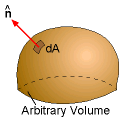Unit Normal Vector

According to the first law of thermodynamics, energy can neither be created nor destroyed. It can only change forms. Similar to the conservation of mass, this concept can be modeled as a set of integral terms, one for the control volume, and one for the control surface. However, unlike the conservation of mass, new energy can be added or subtracted from the system through heat and work. The final integral form of the energy equation for a control volume is given by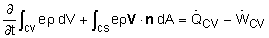where e is the specific total energy (energy per unit mass), V is the velocity of the fluid,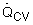is the rate of heat transfer added to the control volume,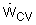is the rate of work transfer (also known as power) done by the control volume, and n is the unit normal vector.

Basically, the energy equation states that:which is equivalent to the first law of thermodynamics.

For steady flow, the first term on the left hand side of the energy equation vanishes. The sign on the quantity V·n depends on the velocity as well as the orientation of the control surface. The unit normal vector is defined as positive when pointing out of the control surface. Hence, the term V·n will be positive when the flow is out of the control volume. This is similar to the momentum integral equation developed previously. For steady flow and constant area (assuming V and n are perpendicular, such as pipe inlet and outlet, the energy equation becomes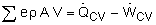Total Energy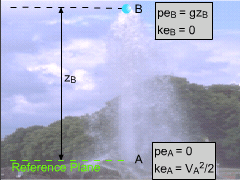Kinetic and Potential Energy

The total energy term, e, needs special explanation. The total energy (E) stored in a system exists in three basic forms: kinetic energy (KE), potential energy (PE), and internal energy (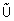) giving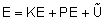The above relation can also be expressed in terms of per unit mass simply by dividing by the mass,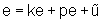The specific kinetic energy is the energy associated with the motion of the system, and is similar to particle energy developed in dynamics. It is given by

ke = V2/2

where V is the velocity of the system (there is no mass since this is per unit mass).

The specific potential energy is the energy associated with the elevation of the system with respect to an arbitrarily chosen reference plane:

pe = gz

where g is the gravitational acceleration, and z is the elevation of the system.

The specific internal energy is the sum of all microscopic forms of energy (e.g., molecular or atomic energy) that a system possesses. Now, the energy equation becomes (recall, dm/dt = ρVA)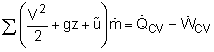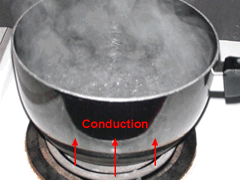Conduction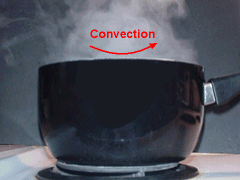Convection

Heat

Heat transfer is energy transfer due to a temperature difference. Heat is always transferred from the high temperature region to the low temperature region. Heat transfer is considered positive when it is added to the control volume, while it is negative when heat escapes from the control volume. When a control volume is said to undergo an adiabatic process, that means heat transfer does not occur (i.e.,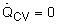).

There are three modes of heat transfer: conduction, convection and radiation. Heat transfer by conduction is mainly through the contact of two bodies, and it can occur in solids, liquids and gases. For example, heat is conducted from the heated stove to the pot, as shown in the figure. Heat transfer by a fluid in motion is referred to as convection. The motion of the fluid can be created artificially (forced convection) or naturally due to density differences (free convection). Heat transfer through electromagnetic waves is called radiation. The energy of the sun reaches the earth by radiation.

Generally, heat is not involved for basic fluid mechanic problems and is generally 0. However, it should be noted viscous effects will generate heat, and they must be included.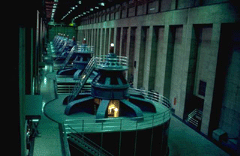Generators at Hoover Dam

Work

Work is the energy transfer associated with a force acting through a distance. Work done by the control volume is considered positive. Examples of work include flow work and shaft work.

When a fluid flows through a control volume, flow work arises due to the pressure forces exerted on the inlets and outlets. The flow work is given by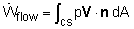where p is the pressure, A is the cross-sectional area, and V is the velocity of the fluid. If the velocity is perpendicular to the surface, dA, and A is constant, then this simplifies to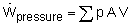The work due to pressure can be both positive or negative depending if the pressure is acting with the flow (negative, n·V = -V) or against the flow (positive).

Shaft work is encountered frequently in engineering applications (e.g., turbines or the power transmission of a car), and it is given by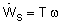where T is the torque of the shaft , and ω is the angular velocity of the shaft. For example, shaft work produced from turbines is converted to electricity through generators in hydroelectric power plants. Power out would be positive, like a turbine.

Combining heat and work terms, the steady state energy equation becomes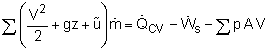with Inlet and Outlet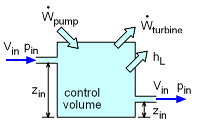with Inlet and Outlet

A common application of the steady state energy equation is for a control volume with inlets and outlets. Using the subscripts 'in' and 'out' to describe flow into the system, respectively, and dividing by g(dm/dt) gives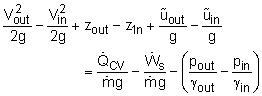The u and Q terms are usually grouped together, and simply labeled as 'head loss', hL,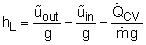This includes all viscous effects such as flow through pipes. Also accounts for piping and inlet/outlet edge energy loss mainly due to viscous flow. It is also useful to split the work as a pump (energy in) and a turbine (energy out). The final form is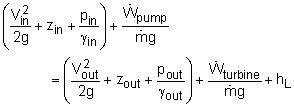where the subscript 'pump' represents energy being supplied into the system and 'turbine' represents energy being removed (to do useful work). Both terms is absolute energy in or out and does not consider the efficiency of the pump or turbine. In a practical application, the internal energy loss of the pump or turbine needs to be taken into account.

This equation assumes just one inlet and one outlet for a control volume, and steady state. This equation is similar to the famous Bernoulli's Equation but includes work and viscous effects.

Practice Homework and Test problems now available in the 'Eng Fluids' mobile app
Includes over 250 problems with complete detailed solutions.
Available now at the Google Play Store and Apple App Store.